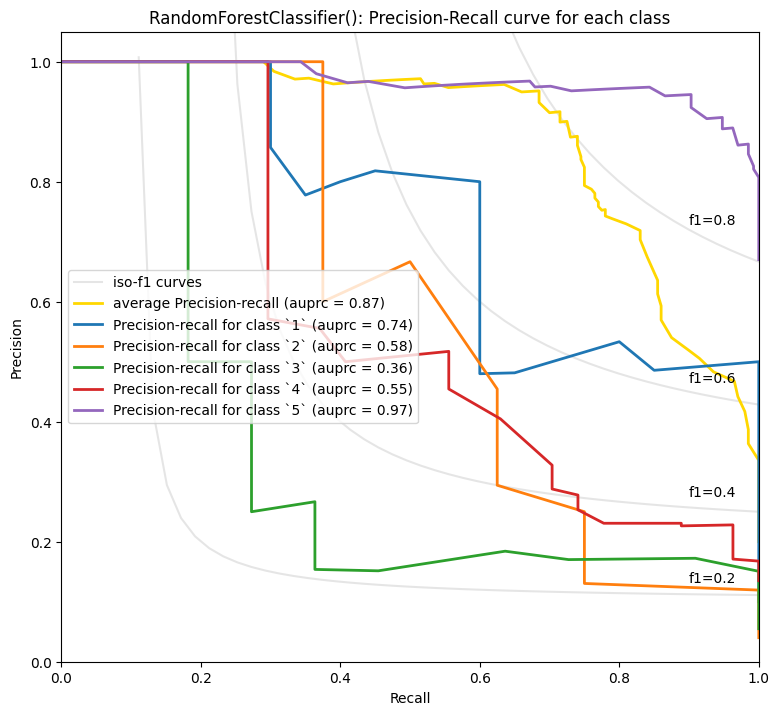# Classification using embeddings

,
Jul 11, 2022
Open in Github

There are many ways to classify text. This notebook shares an example of text classification using embeddings. For many text classification tasks, we've seen fine-tuned models do better than embeddings. See an example of fine-tuned models for classification in Fine-tuned_classification.ipynb. We also recommend having more examples than embedding dimensions, which we don't quite achieve here.

In this text classification task, we predict the score of a food review (1 to 5) based on the embedding of the review's text. We split the dataset into a training and a testing set for all the following tasks, so we can realistically evaluate performance on unseen data. The dataset is created in the Get_embeddings_from_dataset Notebook.

``````# imports
import pandas as pd
import numpy as np
from ast import literal_eval

from sklearn.ensemble import RandomForestClassifier
from sklearn.model_selection import train_test_split
from sklearn.metrics import classification_report, accuracy_score

datafile_path = "data/fine_food_reviews_with_embeddings_1k.csv"

df["embedding"] = df.embedding.apply(literal_eval).apply(np.array)  # convert string to array

# split data into train and test
X_train, X_test, y_train, y_test = train_test_split(
list(df.embedding.values), df.Score, test_size=0.2, random_state=42
)

# train random forest classifier
clf = RandomForestClassifier(n_estimators=100)
clf.fit(X_train, y_train)
preds = clf.predict(X_test)
probas = clf.predict_proba(X_test)

report = classification_report(y_test, preds)
print(report)
``````
```              precision    recall  f1-score   support

1       0.89      0.40      0.55        20
2       1.00      0.38      0.55         8
3       1.00      0.18      0.31        11
4       1.00      0.26      0.41        27
5       0.75      1.00      0.86       134

accuracy                           0.77       200
macro avg       0.93      0.44      0.53       200
weighted avg       0.82      0.77      0.72       200

```

We can see that the model has learnt to distinguish between the categories decently. 5-star reviews show the best performance overall, and this is not too surprising, since they are the most common in the dataset.

``````from utils.embeddings_utils import plot_multiclass_precision_recall

plot_multiclass_precision_recall(probas, y_test, [1, 2, 3, 4, 5], clf)
``````
```RandomForestClassifier() - Average precision score over all classes: 0.87
```Unsurprisingly 5-star and 1-star reviews seem to be easier to predict. Perhaps with more data, the nuances between 2-4 stars could be better predicted, but there's also probably more subjectivity in how people use the inbetween scores.